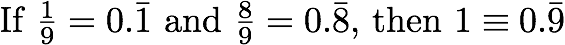# 1 is by definition 0.999999999 9?

Hi there,

I have a question regarding this statement:My question is whether we can say so...

Thank you very much!

OP, the answer is that .999... = 1. It's an equality. They're two expressions that represent the same number.

The reason this is so is that .999... is defined as the infinite sum

9/10 + 9/100 + 9/1000 + ...

This is a geometric series whose sum is 1. This is proven in first-year calculus.

Another way to see it is that there's no distance between the number denoted by .999... and the number denoted by 1. That is, suppose you say, well, .999... is 1/zillion away from1. But I'll just point out that if you take enough 9's, you'll eventually get WITHIN 1/zillion of 1.

So if there's no conceivable positive difference between .999... and 1, then they must represent the same number.

Possible conceptual objections to this reasoning are things like:

* "But how can you have two different expressions for the same number?" Easy. 4 and 2 + 2 are two different expressions for the same number. It happens all the time.

* There must be an "infinitesimal" difference between 1 and .999..." In the standard real number system, there are no infinitesimals. A distance is either zero or positive. Since there's no positive distance between .999... and 1, the distance between them is zero and they're the same number.

Hope this helps. There are discussions of this topic all over the net.

Last edited:

Hurkyl
Staff Emeritus